Mathematics Exam Paper ~ 20781. If f(x) = x2 + kx + 1 for all x and f is an even function then the value of K is equal to
(a) 1 (b) 2 (c) 0 (d) -1

2. If A = [-2, 4) and B = (2, 5] then A ∩ B is
(a) [-2, 5]  (b) (2, 4)  (c) [-2, 2]  (d) (-2, 5)

3. If a = √6, b=2, c = √3 - 1 then B is
(a) 45  (b) 30  (c) 60  (d) 135

4. The equation of diameter of the circle x2 + y2 - 6x + 2y = 0, which passes through the origin is
(a) x + 3y = 0 (b) x - 3y = 0 (c) 3x + y = 0 (d) 3x - y = 0

5. The locus is known as parabola if the eccentricity (e) is
(a) equal to 1 (b) less than 1 (c) more than 1 (d) equal to zero

6.then the projection of b on a is
(a) 3   (b) 4    (c) 5    (d) 6

7. Form the group of 10 items ∑x = 452, ∑x2 = 24720 and mode = 43.7. Then the coefficient of skewness if
(a) -0.077  (b) 0.077   (c) 0.079    (d) - 0.079

8. The differentiating coefficient of cos-1x + sin-1x is equal to
(a) π/2  (b) 2/√(1-x2)    (c) 0  (d) none of these

9. The value of(a) 3x - 7 ln |x+2| + c    (b) 3x + 7 ln|x+2| + c   (c) 3x - 7 ln|3x-1| + c  (d) 3x + 7 ln|3x-1| + c

10. A body is dropped from tower of height 78.4 m. Then the time to reach the ground is
(a) 6s  (b) 4s   (c) 3s   (d) 2s

OR

The demand function P = 12 - 0.5Q then the marginal revenue is
(a) 12-Q  (b) 12-PQ  (c) 12+Q  (d) 12 + PQ

11. . How many iterations do you need to get the approximate root of an equation f(x) = 0 by by bisection method if you start with initial guesses a = 1 , b = 2 and error tolerance 10-4?
(a) 12   (b) 10   (c) 2  (d) 14

"Group B" [ 5 × 8 = 40 ]

12. (a) If p and q are two statements then prove that:  p ⇒ q ≡ (~p v q) 
(b). Is the function f: N→N defined by f(x) = 3x bijective? 

13. (a) Find the square root of the complex number: -5 + 12i. 
(b) If ax = by = cz and a, b, c are in G.P. then show that x, y and z are in H.P. 

14. (a) In any triangle ABC, prove that(b) If two pair of straight lines represented x2 - 2axy - 3y2 = 0 and x2 - 2bxy - y2 = 0 are such that each pair bisects the angle between the other pair, prove that ab + 2 = 0. 

15. (a) Calculate the Karl Pearson's coefficient of skewness from the following data: 

 Profit below 80 below 90 below 100 below 110 below 120 No. of cost 12 30 65 107 130

b. A class consists of 30 boys and 20 girls. If two students are chosen at random, then find the probability that 
i. one is boy another is girl
ii. both are girls

16. a. Evaluate:b. Write the conditions that the graph of the function y = f(x) defined in an interval ( a, b ) is concave upward and concave downward. Find the maximum and minimum values of curve: y = 4x3 - 6x2 - 9x + 1 on the interval (-1,2). Also, find the points of inflection.  

17. a. Integrate: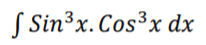b. What does an indefinite integral mean? How does it differ from definite integral? Obtain the area bounded by the curves y = 4x and x = 4y.  

18. Use simplex method to find the optimal solutions of the LPP:
Maximize: F = 2x + 5y subject to the constraints.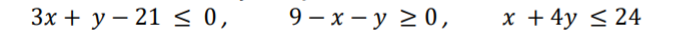where x,y ≥ 0.  

19. a. Resolve a force 30N into two components making angles 30° and 45° with its direction. 
b. A stone is dropped into a well reaches the water with velocity 49m/s and sound of striking water is heard in 5⅓ seconds after it is let fall. Find the velocity of the sound. 
OR
19. a. If the marginal cost of product is given by C' (x) = 36 - 20x + 6x2, where x is the number  of units and initial cost is Rs 20, then find the total cost function and average cost function. 
b. The demand function for a good is given as Q = 65 - 5P. Fixed the costs are Rs 30 and each unit produced costs an additional Rs 2. 
i. Write the equations for total revenue and total costs interms of Q.
ii. find the break-even points algebraically.
iii. Graph the total revenue and total costs and hence estimate the break-even point.

"Group C" [ 8 × 3 = 24 ]

20. a. Find the domain and the range of the function: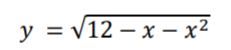[ 4 + 4 = 8 ]

b. Draw the graph of the function including different characteristics: y = 2Sinx ( 0 ≤ x ≤ 2π )

21. a. Given a = √3 + 1, b = √6, c = √3 -1, solve the triangle ABC.     [ 3 + 3 + 2 = 8 ]
b. Show that the angle between the tangents to the parabola y2 = 4x and x2 = 4y at their points of intersection other than origin is tan-1¾.
c. Prove vectorially that: a2 = b2 + c2 - 2bcCosA

22. a. Find from the first principles the derivative of: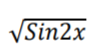[ 4 + 2 + 2 = 8 ]
b. A spherical balloon is inflated at the rate of 20cm3/sec. Find the rate of increasing its surface area when the radius is 8cm.
c. Evaluate: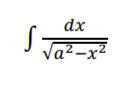⁕⁕⁕